# Conservative Force

Before we get into energy in earnest, let’s return to force for once.

Let’s group the forces we’ve learned so far in terms of work!

## Let’s transport objects, Part 1

The figure below shows a room viewed from directly above.
An object at A in the figure is pulled with a constant force of 20 N to move at constant velocity to B.

In this case, we want to consider whether the amount of work done by the kinetic friction changes depending on the path taken when transporting from A to B.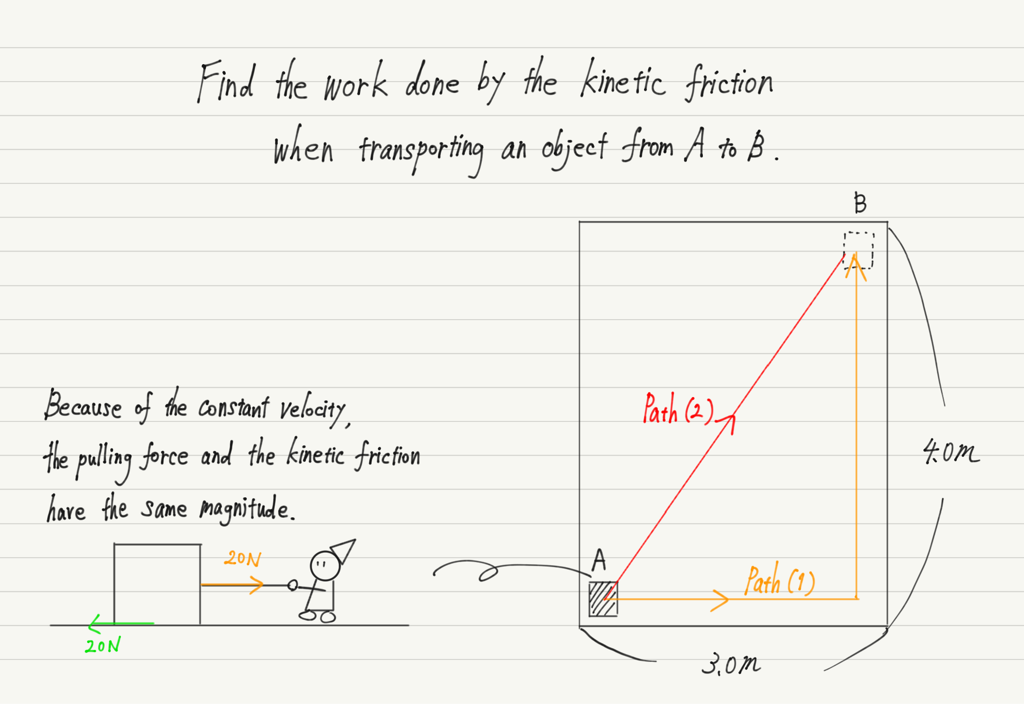Now let’s do the calculations.

First, the total distance traveled from A to B is 3.0 m + 4.0 m = 7.0 m for the path of (1).
Therefore, the work done by the kinetic friction is 20 N × 7.0 m × (-1) = -140 J.

If you do not understand the calculation of this work, review it.

https://www.yukimura-physics.com/entry/dyn-f23

Since the path of (2) carries on the diagonal, the distance traveled is 5.0 m (from the Pythagorean theorem).
Therefore, the work done by the kinetic friction is 20 N × 5.0 m × (-1) = -100 J.

Comparing the results of the above calculations, we can see that the work done by the kinetic friction varies depending on which path is taken.
Since the kinetic friction is a force that hinders motion, the shorter the distance, the less hindered it is.

## Let’s transport objects, Part 2

Next, consider the work done by gravitational force in moving an object from a high place to a low place.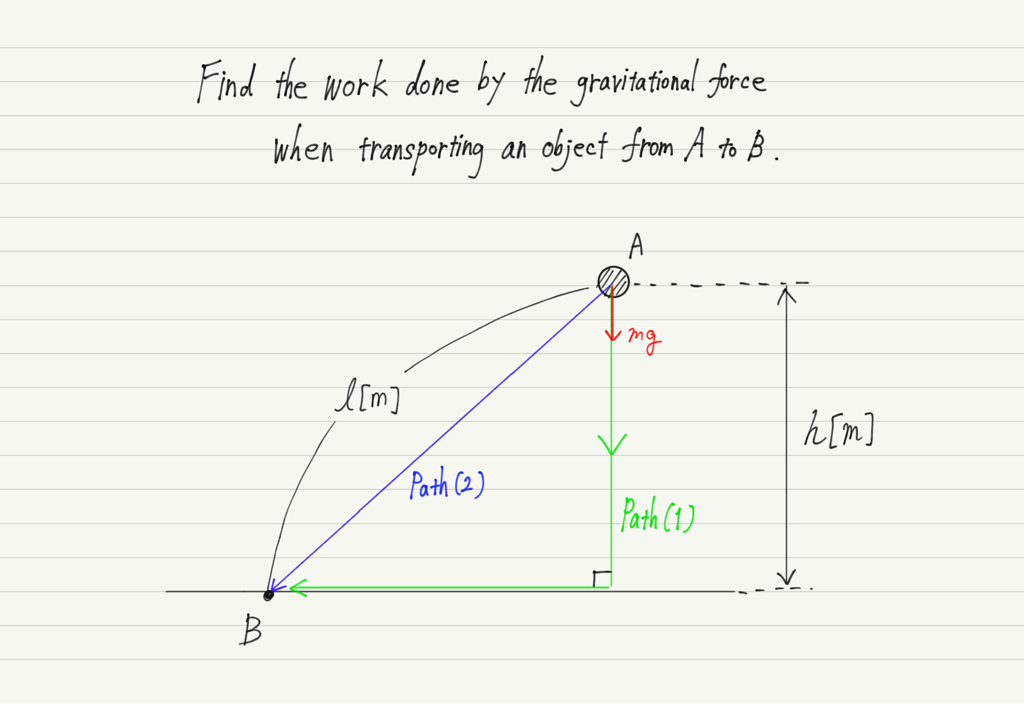The path of (1) is moved first directly down and then directly sideways.
When directly down, the magnitude of gravitational force is mg and the distance is h, so the work is mgh.

When directly sideways, the direction of gravitational force and the direction of movement are perpendicular, so the work done by gravitational force is zero.

Thus, the overall work for the path of (1) is mgh + 0 = mgh.

Next, for the path of (2), note that the direction of gravitational force and the direction of movement are not in the same direction, opposite, or perpendicular.
If the force is oblique to the direction of movement, decompose the force to find the component in the direction of movement and then calculate the work.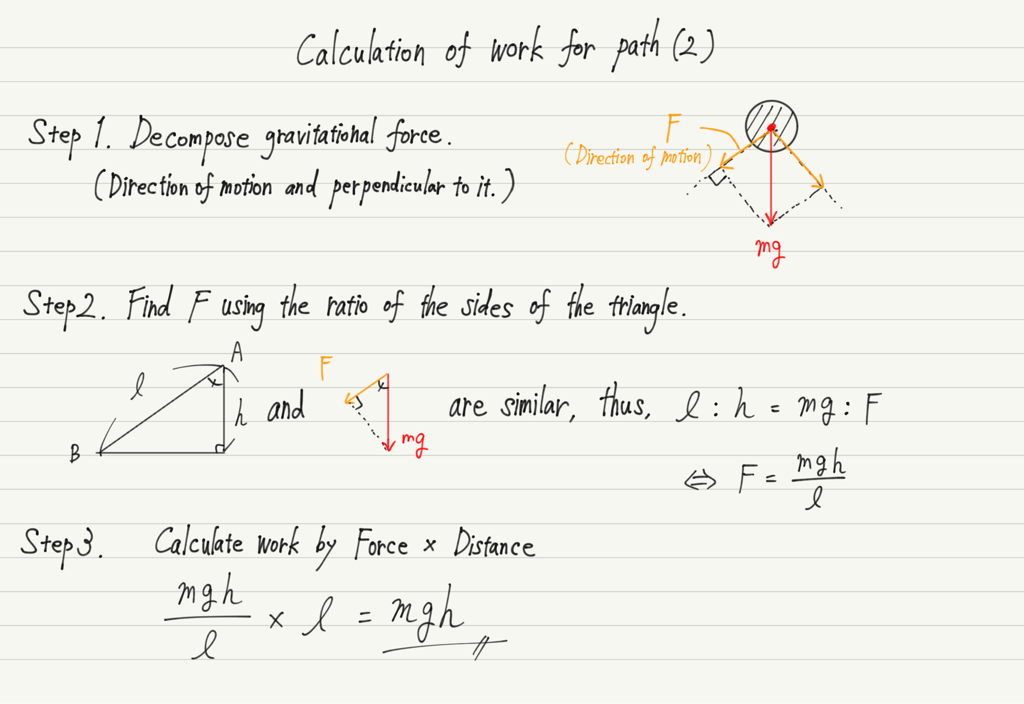https://www.yukimura-physics.com/entry/dyn-f17

Now, if we look at the result of the above calculation, we see that the work done by gravitational force is mgh even in the path of (2)!

In fact, it is known that the work done by gravitational force is always constant, no matter which path an object is carried.
In the example above we calculated only two ways, but no matter how complicated the path from A to B, the work done by gravitational force is always mgh!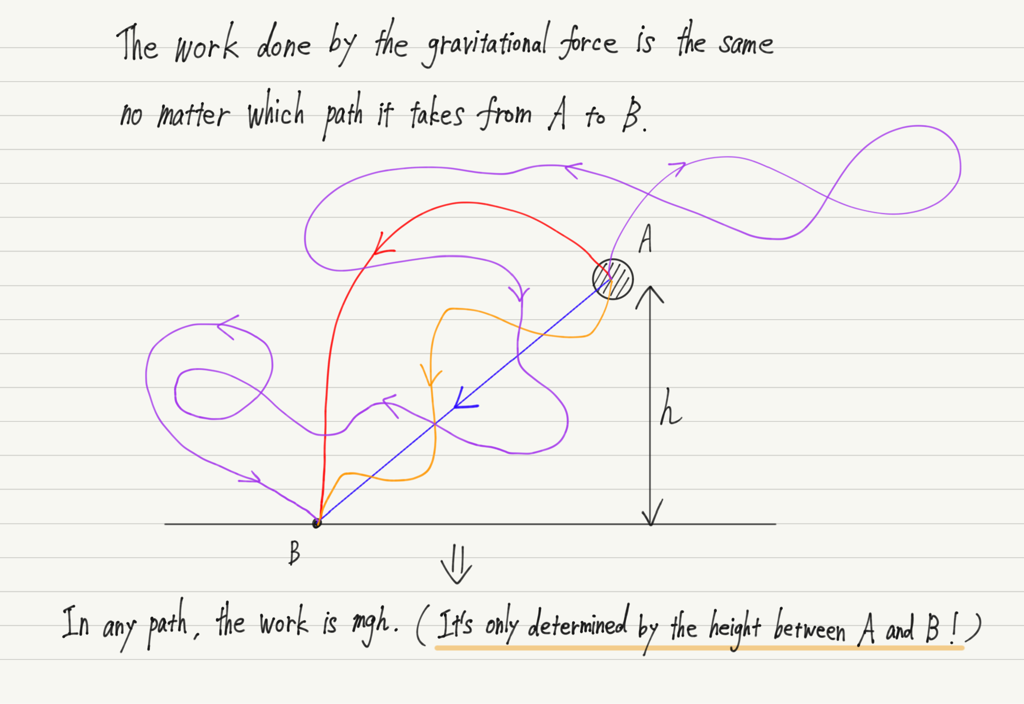## What is conservative force?

What we have learned from the above calculations is that there are two types of forces: a force whose work value changes depending on the path it takes, and a force whose work value does not change along any path.

A force like gravitational force that does not change its work no matter which path it takes is called a “Conservative force”.

In other words, if you know the starting point and the arrival point, that’s all you need to know to calculate the work!
(For example, the work done by gravitational force can be calculated with only the difference in height h between two points.)

On the other hand, a force such as kinetic friction, whose work changes depending on the path, is called “Nonconservative force”.

The property of conservative forces is very powerful in calculating work.
You absolutely must remember which forces are conservative!

## Summary of this lecture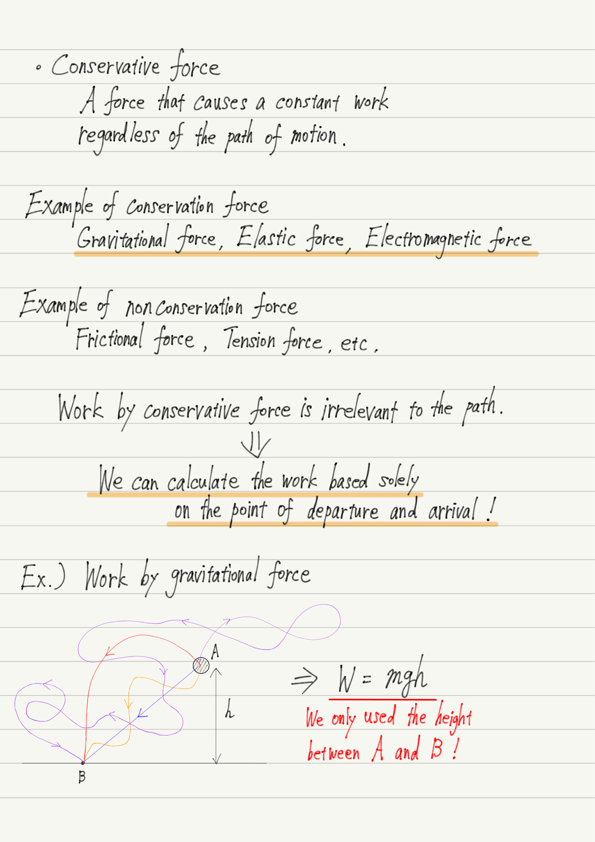## Next Time

Next time we’ll talk kinetic energy!

https://www.yukimura-physics.com/entry/dyn-f26

error: Content is protected !!